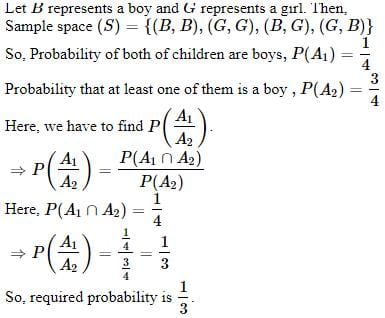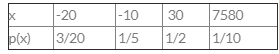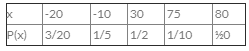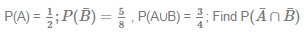# Test: Probability And Expected Value By Mathematical Expectation- 6

## 40 Questions MCQ Test Quantitative Aptitude for CA CPT | Test: Probability And Expected Value By Mathematical Expectation- 6

Description
Attempt Test: Probability And Expected Value By Mathematical Expectation- 6 | 40 questions in 40 minutes | Mock test for CA Foundation preparation | Free important questions MCQ to study Quantitative Aptitude for CA CPT for CA Foundation Exam | Download free PDF with solutions
QUESTION: 1

Solution:
QUESTION: 2

### A family has 2 children. The probability that both of them are boys if it is known that one of them is a boy

Solution:QUESTION: 3

### The Probability of the occurrence of a no. greater then 2 in a throw of a die if it is known that only even nos. can occur is

Solution:

Total events are 6
Possibility of Greater than 2 is 4 i.e. 3, 4, 5, 6
Hence, Probability of the occurrence of a no. greater then 2 will be 4/6

=2/3

QUESTION: 4

A player has 7 cards in hand of which 5 are red and of these five 2 are kings. A card is drawn at random. The probability that it is a king, it being known that it is red is

Solution:
QUESTION: 5

In a class 40 % students read Mathematics, 25 % Biology and 15 % both Mathematics and Biology. One student is select at random. The probability that he reads Mathematics if it is known that he reads Biology is

Solution:
QUESTION: 6

In a class 40 % students read Mathematics, 25 % Biology and 15 % both Mathematics and Biology. One student is select at random.The probability that he reads Biology if he reads Mathematics

Solution:
QUESTION: 7

Probability of throwing an odd no with an ordinary six faced die is

Solution:
QUESTION: 8

For a certain event A ,P (A) is equal to

Solution:
QUESTION: 9

When none of the outcomes is favourable to the event then the event is said to be

Solution:
QUESTION: 10

The probability of selecting sample of size 'n' out of a population of size N by simple random sampling with replacement is:

Solution:
QUESTION: 11

If P(A) = 0.45, P(B) = 0.35 and P(A &  B) = 0.25, then P(A/B)=?

Solution:
QUESTION: 12

Find the probability of drawing a spade on each of two consecutive draws from a well shuffled pack of cards, without replacement

Solution:

in a pack of card there are total  52 Cards

Probability that two consecutive cards drawn  from a well shuffled pack of cards are spade = ¹³C₂ / ⁵²C₂

= 13 * 12 / 52 * 51

= 12 / 4 * 51

= 3/51

= 1/17

other ways

probability of Choosing 1st card as spade = (13/52) = 1/4

Probability of choosing 2nd spade card = 12/51  = 4/17

(1/4)(4/17)  = 1/17

QUESTION: 13

If a pair of dice is thrown then the probability that the sum of the digit is neither 7 nor 11 is _____.

Solution:
QUESTION: 14

A player tosses 3 fair coins. He wins Rs.5 if three appear, Rs.3 if two heads appear, Rs.1 if one head occurs. On the other hand, he losses Rs.15 if 3 tails occur. Find expected gain of the player.

Solution:
QUESTION: 15

The probability of a cricket term winning match at Kanpur is 2/5 and hosing match at Delhi is 1/7 what is the Probability of the term winning atleast one match?

Solution:
QUESTION: 16

Two coins are tossed simultaneously. Find the probability of getting exactly one head.

Solution:
QUESTION: 17

For any two events A1, A2 letP(A1) = 2/3,P(A2) = 3/8 and P(A1 ∩ A2) =1/4 then A1, Aare:

Solution:
QUESTION: 18

Find the expected value of the following probability distribution1/20

Solution:
QUESTION: 19

The odds against A solving a certain problem are 4 to 3 and the odds in favour of B solving the same problem are 7 to 5. What is the probability that the problem will be solved if they both try?

Solution:
QUESTION: 20

A box contains 2 red, 3 green and 2 blue balls. Two bails are drawn at random. What is the probability that none of the balls drawn is blue?

Solution:
QUESTION: 21

The odds that a book will be favourable received by 3 independent received by 3 independent reviewers are 5 to 2, 4 to 3 and 4 to 4 respectively. What is the probability that out of 3 reviewers a majority will be favourable?

Solution:
QUESTION: 22

An urn contains 2 red and 1 green balls. nother urn contains 2 red and 2 green balls. An urn was selected at random and then a ball was drawn from it. If it was found to be red then the probability that it has been drawn from urn one is ______.

Solution:
QUESTION: 23

Find the expected value of the following probability distributionSolution:
QUESTION: 24

A bag contains 6 red balls and some blue balls. If the probability of drawing a blue ball form the bag is twice that of a red ball, find the number of blue balls in the bag

Solution:
QUESTION: 25

A company employed 7 CA's, 6 MBA's and 3 Engineer's. In how many ways the company can form a committe, if the committee has  two members of each type.

Solution:
QUESTION: 26

In a packet of 500 pens, 50 are found to be defective. A pen is selected at random. Find the probability that it is non defective.

Solution:
QUESTION: 27

A bag contains 5 Red balls, 4 Blue Balls and 'm' Green Balls. If the random probability of picking two green balls is 1/7. What is the no. of green Balls (m).

Solution:
QUESTION: 28

Four married couples have gathered in a room. Two persons are selected at random amongst them, find the probability that selected persons are a gentleman and a lady but not a Couple.

Solution:
QUESTION: 29

The odds against A solving a certain problem are 4 to 3 and the odds in favour of B solving the same problem are 7 to 5. What is the probability that the problem will be solved if they both try?

Solution:
QUESTION: 30

One Card is drawn from pack of 52, what is the probability that it is a king or a queen?

Solution:
QUESTION: 31

A player tosses two fair coins, he wins Rs.5 of 2 head appear, Rs.2 if one head appears and Rs.1 if no head occurs. Find his expected amount of winning.

Solution:
QUESTION: 32

Arun & Tarun appear for an interview for two vacancies. The probability of Arun's selection is 1/3 and that of Tarun's selection is 1/5. Find the probability that only one of them will be selected.

Solution:
QUESTION: 33

If 15 persons are to be seated around 2 round tables, one occupying 8 persons and another 7 persons.Find the number of ways in which they can be seated.

Solution:
QUESTION: 34

The probability of Girl getting scholarship is 0.6 and the same probability for Boy is 0.8. Find the probability that at least one of the categories getiing scholarship.

Solution:
QUESTION: 35

A coin is tossed 5 times, what is the probability that exactly 3 heads will occur.

Solution:
QUESTION: 36

Two dice are thrown together. Find the probability of getting a multiple of 2 on one dice and multiple of 3 on the other.

Solution:

Two dice are thrown simultaneously

S={(1,1),(1,2),(1,3),(1,4),(1,5), (1,6)

(2,1)(2,2)(2,3)(2,4)(2,5)(2,6)

(3,1)(3,2)(3,3)(3,4)(3,5)(3,6)

(4,1)(4,2)(4,3)(4,4)(4,5)(4,6)

(5,1)(5,2)(5,3)(5,4)(5,5)(5,6)

(6,1)(6,2)(6,3)(6,4)(6,5)(6,6)}

n(s)=36

Event A : getting multiple of 2 on one die and multiple of 3 on other die
A={(2,3)(2,6)(4,3)(4,6) (6,3)(6,6)(6,2)(6,4)(3,2)(3,6)}
n(A)=10
p(A)=n(A)/n(s)
=10/36
=5/18

QUESTION: 37

Two unbiased dice are thrown. The Expected value of the sum of numbers on the upper side is;

Solution:
QUESTION: 38

Let A and B two events in a sample space S such thatSolution:
QUESTION: 39

A card is drawn out of a standard pack of 52 cards. What is the probability of drawing a king or red colour?

Solution:
QUESTION: 40

In how many ways a team of 5 can be made out of 7 Boys and 8 Girls, if 2 Girls are compulsory to form a Team.

Solution:Use Code STAYHOME200 and get INR 200 additional OFF Use Coupon Code